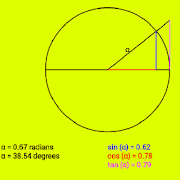# SINE, COSINE AND TANGENTEveryone
92
The GRAPHICS option shows the sine, cosine and tangent of an angle in a circle of radius equal to one.

You can use this graphic as an interactive trigonometric tables by touching on the circle or by entering the angle value in degrees.

The RIGHT TRIANGLE option describes how to calculate sine and cosine in a right triangle and asks you to estimate some values.

The option GRAPHING FUNCTIONS accesses the representation of the trigonometric functions on Cartesian coordinate axes (abscissa and ordinate axes).

You can select the function that you want to represent (sine, cosine and tangent), modify its amplitude, period or midline, obtaining its representation and the display of the corresponding function (for example: f (x) = 1 + 3 sine 2x ).

It also has several HELPS and visualization of the most relevant IDENTITIES of trigonometric functions.
Collapse

## Reviews

Review policy and info
3.4
92 total
5
4
3
2
1

## What's New

- Graphing trigonometric functions

- Amplitude, period and midline of graphs

- Trigonometric function identities
Collapse

Updated
February 8, 2017
Size
3.0M
Installs
10,000+
Current Version
Y2017
Requires Android
4.0.3 and up
Content Rating
Everyone
Permissions
Offered By